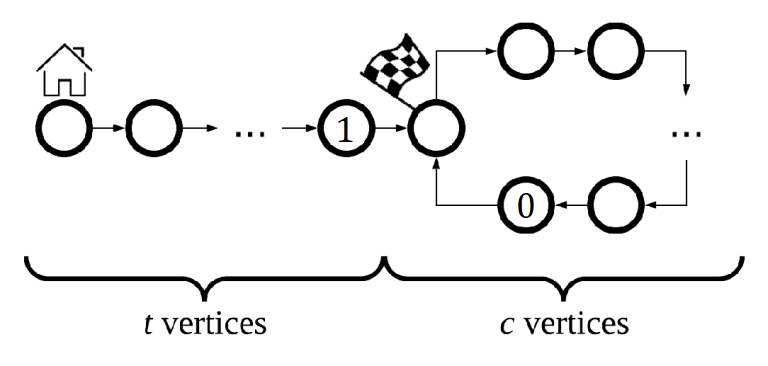# Codeforces Round #545 (Div. 2) 题解

## A. Sushi for Two

### 题意

$2\le n\le 100\ 000$

• 枚举

## B. Circus

### 题意

$2\le n\le 5\ 000$

### 题解

$O(n^2)$ 的做法其实有些冗余，所以找了一种好理解的 $O(n)$ 算法。

• 枚举
• 构造

## C. Skyscrapers

### 题意

$1\le n,m\le 1\ 000$

• 排序
• 贪心

## D. Camp Schedule

### 题意

$1\le |s|,|t|\le 500\ 000$

• KMP
• 构造

## E. Museums Tour

### 题意

$1\le d\le 50$

$1\le n\le 100\ 000$

$1\le m\le 100\ 000$

### 题解

• 在连边后的图上，如果原图中的点 $[i,j]$ 向 $[i,k]$ 有连边，那么 $[i,k]$ 一定向 $[i,j]$ 有连边。因此在路径上可能出现的原图同一个点的新点都会被缩到同一个强连通分量里。

• tarjan
• 拓扑排序

## F. Cooperative Game

### 题意$1\le t,c,(t+c)\le 1\ 000$

### 题解• Floyd判环
• 交互

### 说点什么Subscribe

/* */